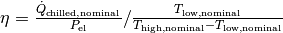# Compression heat pump and chiller¶

Simple calculations for compression heat pumps and chillers.

## Scope¶

This module was developed to provide COP calculations based on temperatures for energy system optimizations with oemof.solph.

A time series of pre-calculated COPs can be used as input for a transformer (an oemof.solph component) in an energy system optimization. Discover more possibilities to use this module with our examples: https://github.com/oemof/oemof-thermal/tree/dev/examples

## Concept¶

Compression heat pumps and chillers increase the temperature of a flow using a compressor that consumes electric power. The inlet heat flux comes from a low temperature source (T_low) and the outlet has the temperature level of the high temperature sink (T_high). The same cycle can be used for heating (heat pump) or cooling (chiller).Fig.1: The heat pump cycle and its two temperature levels.

The efficiency of the heat pump cycle process can be described by the Coefficient of Performance (COP). The COP describes the ratio of useful heat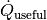(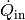or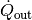) per electric work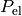consumed: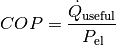The Carnot efficiency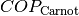describes the maximum theoretical efficiency (ideal process). It depends on the temperature difference between source and sink: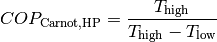for heat pumps andfor chillers.

To determine the COP of a real machine a scale-down factor (the quality grade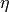) is applied on the Carnot efficiency: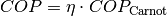with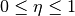Typical values of quality grades are 0.4 for air-source heat pumps, 0.55 for ground-source (“brine-to-water”) heat pumps using a ground heat exchanger, and 0.5 for heat pumps using groundwater as source. 

For high temperature heat pumps Arpagaus finds quality grades between 0.4 and 0.6. 

Fig.2 illustrates how the temperature difference affects the COP and how the choice of the quality grade allows to model different types of heat pumps.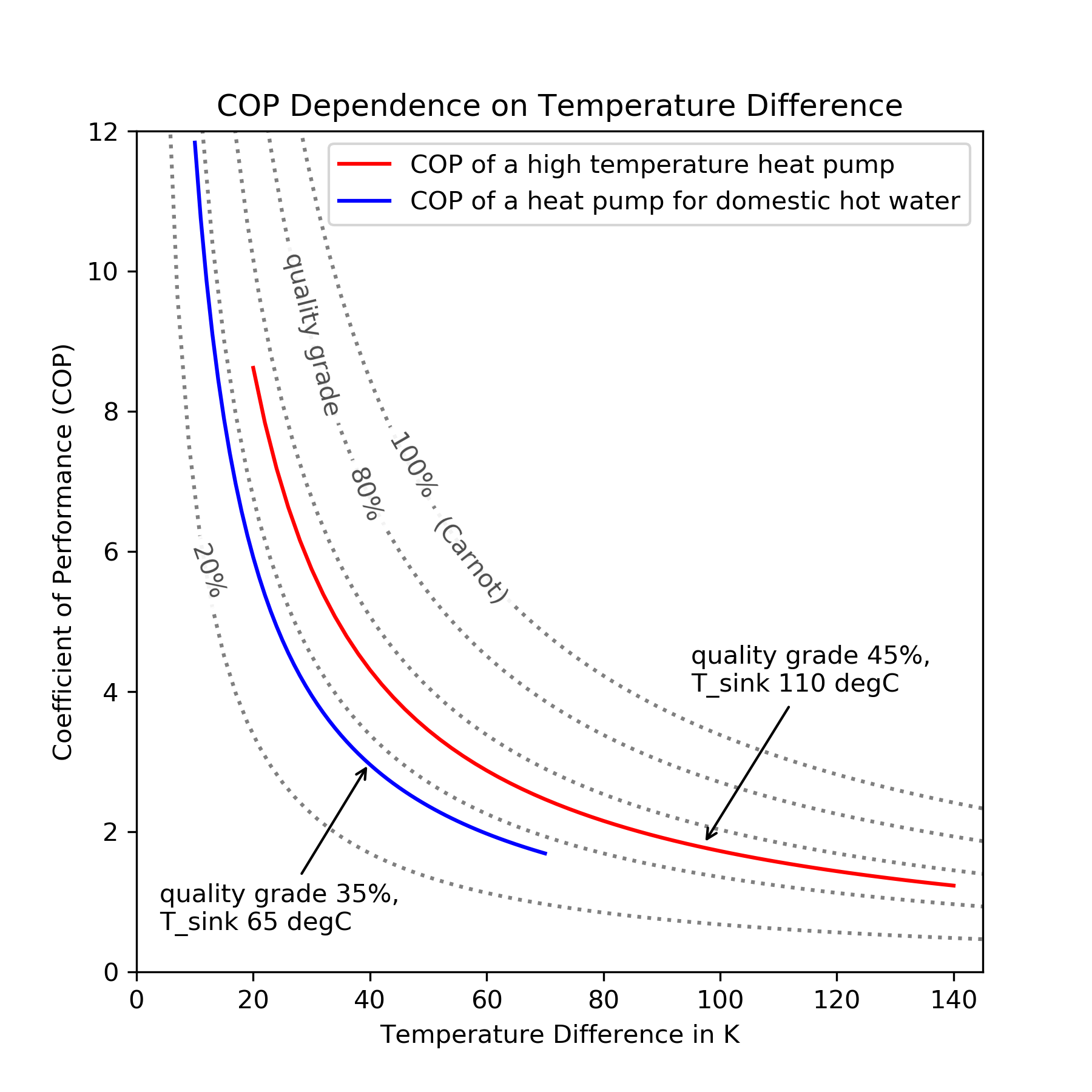Fig.2: COP dependence on temperature difference (Output of example cop_dependence_on_temperature_difference.py).

## Usage¶

These arguments are input to the functions:

symbol attribute explanation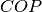cop Coefficient of Performance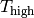temp_high Temperature of the high temp. heat reservoir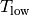temp_low Temperature of the low temp. heat reservoirquality_grade Quality grade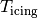temp_threshold_icing Temperature below which icing occurs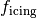factor_icing COP reduction caused by icing

The Coefficient of Performance (COP) is calculated using calc_cops()

COP = calc_cops(temp_high,
temp_low,
temp_threshold_icing,
factor_icing,
mode)


mode=’heat_pump’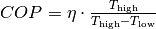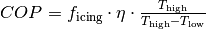mode=’chiller’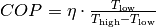The maximum cooling capacity can be calculated using calc_max_Q_dot_chill().

Q_dot_chill_max = calc_max_Q_dot_chill(nominal_conditions, cops)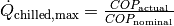The maximum heating capacity can be calculated using calc_max_Q_dot_heat()

Q_dot_heat_max = calc_max_Q_dot_heat(nominal_conditions, cops)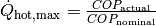The quality grade at nominal point of operation can be calculated using calc_chiller_quality_grade()

Do NOT use this function to determine the input for calc_cops()!

quality_grade = calc_chiller_quality_grade(nominal_conditions)